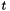## Apophenia

Tests & diagnostics

Here is the model for all hypothesis testing within Apophenia:

• Calculate a statistic.
• Describe the distribution of that statistic.
• Work out how much of the distribution is (above|below|closer to zero than) the statistic.

There are a handful of named tests that produce a known statistic and then compare to a known distribution, like apop_test_kolmogorov or apop_test_fisher_exact. For traditional distributions (Normal,,), use the apop_test convenience function.

In especially common cases, like the parameters from an OLS regression, the commonly-associatedtest is included as part of the estimation output, typically as a row in the info element of the output apop_model.

To give another example of testing, here is a function that was briefly a part of Apophenia, but seemed a bit out of place. Here it is as a sample:

// Input: any vector, which will be normalized in place. Output: 1 - the p-value
// for a chi-squared test to answer the question, "with what confidence can I
// reject the hypothesis that the variance of my data is zero?"
double apop_test_chi_squared_var_not_zero(gsl_vector *in){
Apop_stopif(!in, return NAN, 0, "input vector is NULL. Doing nothing.");
apop_vector_normalize(in, .normalization_type='s');
double sum=apop_vector_map_sum(in, gsl_pow_2);
return gsl_cdf_chisq_P(sum, in->size);
}

Or, consider the Rao statistic,whereis a model's likelihood function andits information matrix. In code:

apop_data * infoinv = apop_model_numerical_covariance(data, your_model);
apop_data * score = &(apop_data*){.vector=apop_numerical_gradient(data, your_model)};
apop_data * stat = apop_dot(apop_dot(score, infoinv), score);

Given the correct assumptions, this is, whereis the dimension of, so the odds of a Type I error given the model is:

double p_value = apop_test(stat, "chi squared", beta->size);

Generalized parameter tests

But if your model is not from the textbook, then you have the tools to apply the above three-step process to the parameters of any apop_model.

• Model parameters are a statistic, and you know that apop_estimate(your_data, your_model) will output a model with a parameters element.
• apop_parameter_model will return an apop_model describing the distribution of these parameters.
• We now have the two ingredients to send to apop_cdf, which takes in a model and a data point and returns the area under the data point.

Defaults for the parameter models are filled in via bootstrapping or resampling, meaning that if your model's parameters are decidedly off the Normal path, you can still test claims about the parameters.

The introductory example in A quick overview ran a standard OLS regression, whose output includes some standard hypothesis tests; to conclude, let us go the long way and replicate those results via the general apop_parameter_model mechanism. The results here will of course be identical, but the more general mechanism can be used in situations where the standard models don't apply.

The first part of this program is identical to the introductory program, using ss08pdc.csv if you have downloaded it as per the instructions in A quick overview, or a simple sample data set if not. The second half executes the three steps uses many of the above features: one of the inputs to apop_parameter_model (which row of the parameter set to use) is sent by adding a settings group, we pull that row into a separate data set using Apop_r, and we set its vector value by referring to it as the -1st element.

#include <apop.h>
#include <unistd.h>
int main(void){
char *datafile = (access("ss08pdc.csv", R_OK)!=-1) ? "ss08pdc.csv" : "data";
apop_text_to_db(.text_file=datafile, .tabname="dc");
apop_data *data = apop_query_to_data("select log(pincp+10), agep, sex "
"from dc where agep+ pincp+sex is not null and pincp>=0");
apop_model_show(est);
Note that the procedure did not assume the model parameters had a certain form. It queried the model for the distribution of parameter agep, and if the model didn't have a closed-form answer then a distribution via bootstrap would be provided. Then that model was queried for its CDF. [The procedure does assume a symmetric distribution. Fixing this is left as an exercise for the reader.] For a model like OLS, this is entirely overkill, which is why OLS provides the basic hypothesis tests automatically. But for models where the distribution of parameters is unknown or has no closed-form solution, this may be the only recourse.